# The electric field inside a parallel-plate capacitor points directly from the positive plate towards the negative...

The electric field inside a parallel-plate capacitor points directly from the positive plate towards the negative plate in straight lines. Describe what the equipotential lines must look like in a parallel plate capacitor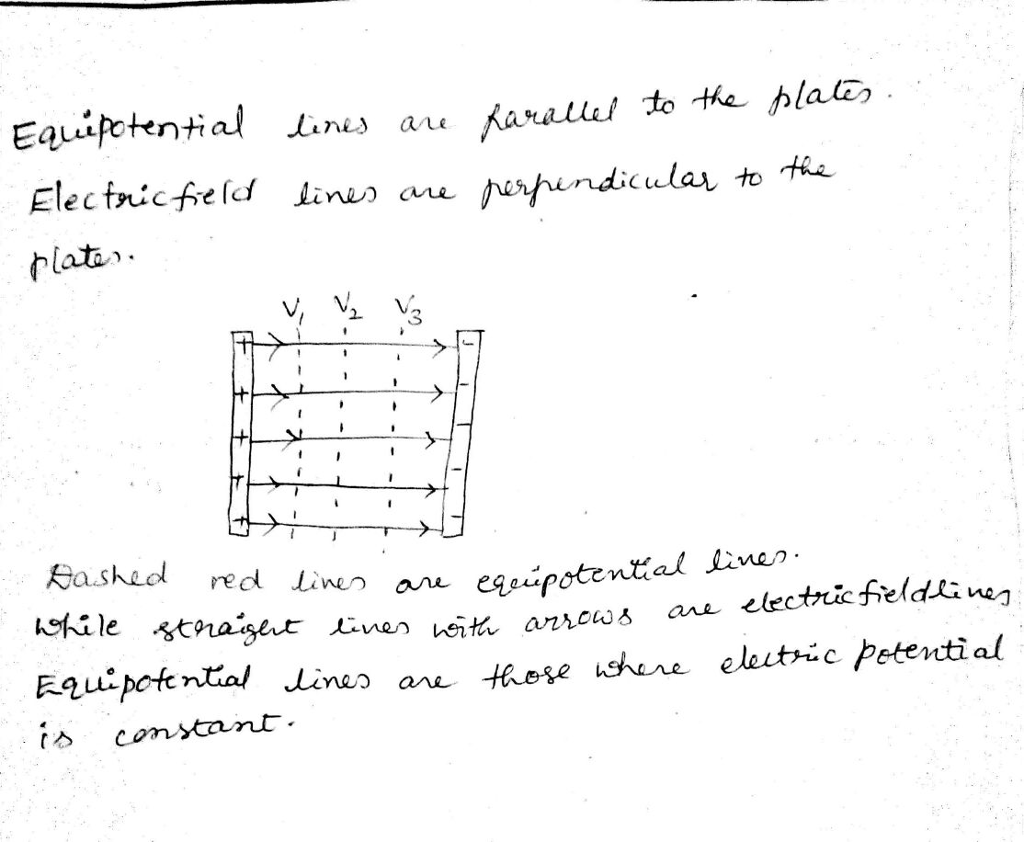##### Add Answer to: The electric field inside a parallel-plate capacitor points directly from the positive plate towards the negative...
Similar Homework Help Questions
• ### The electric field strength is 2.20×104 N/C inside a parallel-plate capacitor with a 1.20 mm spacing....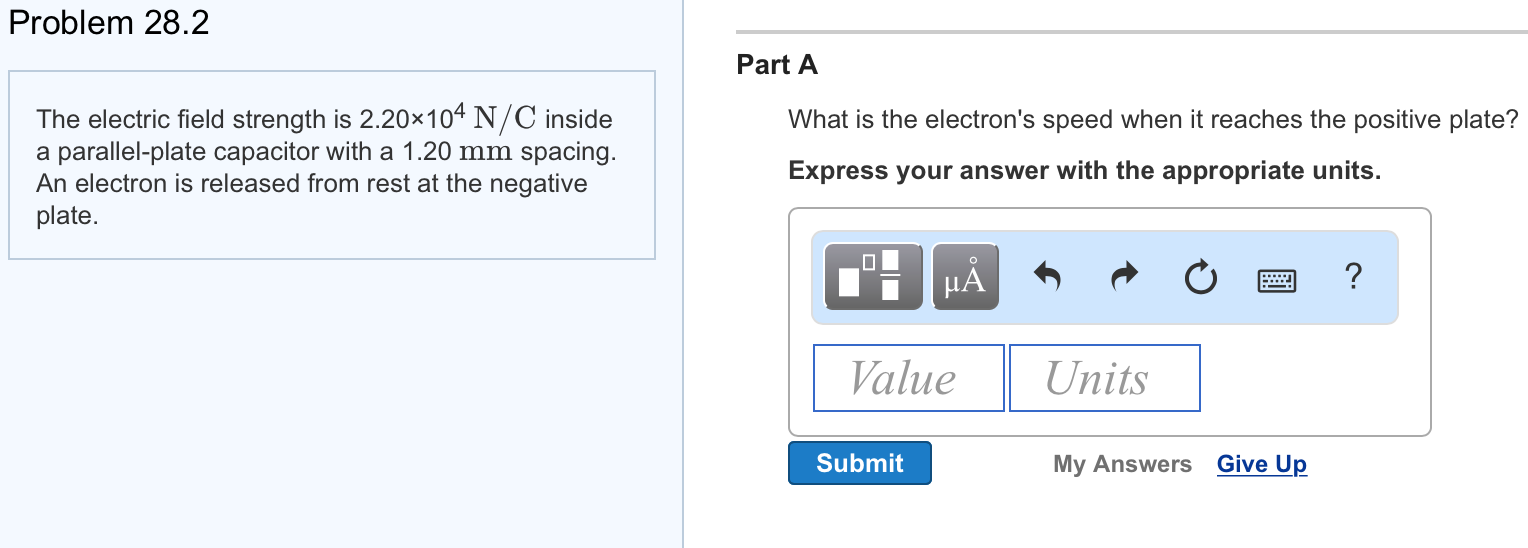The electric field strength is 2.20×104 N/C inside a parallel-plate capacitor with a 1.20 mm spacing. An electron is released from rest at the negative plate. Problem 28.2 Part A The electric field strength is 2.20x104 N/C inside a parallel-plate capacitor with a 1.20 mm spacing. An electron is released from rest at the negative plate. What is the electron's speed when it reaches the positive plate? Express your answer with the appropriate units alueUnits Submit My Answers Give Up

• ### The electric field strength is 6,000 V/m inside a parallel-plate capacitor with a 0.5 mm spacing....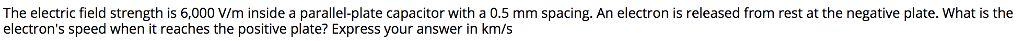The electric field strength is 6,000 V/m inside a parallel-plate capacitor with a 0.5 mm spacing. An electron is released from rest at the negative plate. What is the electron's speed when it reaches the positive plate? Express your answer in km/s

• ### The electric field strength is 5.20×104 N/C inside a parallel-plate capacitor with a 2.40 mm spacing....

The electric field strength is 5.20×104 N/C inside a parallel-plate capacitor with a 2.40 mm spacing. A proton is released from rest at the positive plate. Part A What is the proton's speed when it reaches the negative plate?

• ### An electron is released from rest at the negative plate of a parallel plate capacitor and...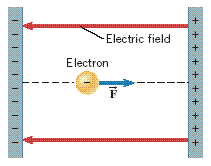An electron is released from rest at the negative plate of a parallel plate capacitor and accelerates to the positive plate (see the drawing). The plates are separated by a distance of 1.7 cm, and the electric field within the capacitor has a magnitude of 2.7 x 106 V/m. What is the kinetic energy of the electron just as it reaches the positive plate? The figure shows a vertical plate on the left that is negatively charged and another vertical...

• ### The electric field strength is 2.00 times 10^4 N/C inside a parallel-plate capacitor with a 0.500...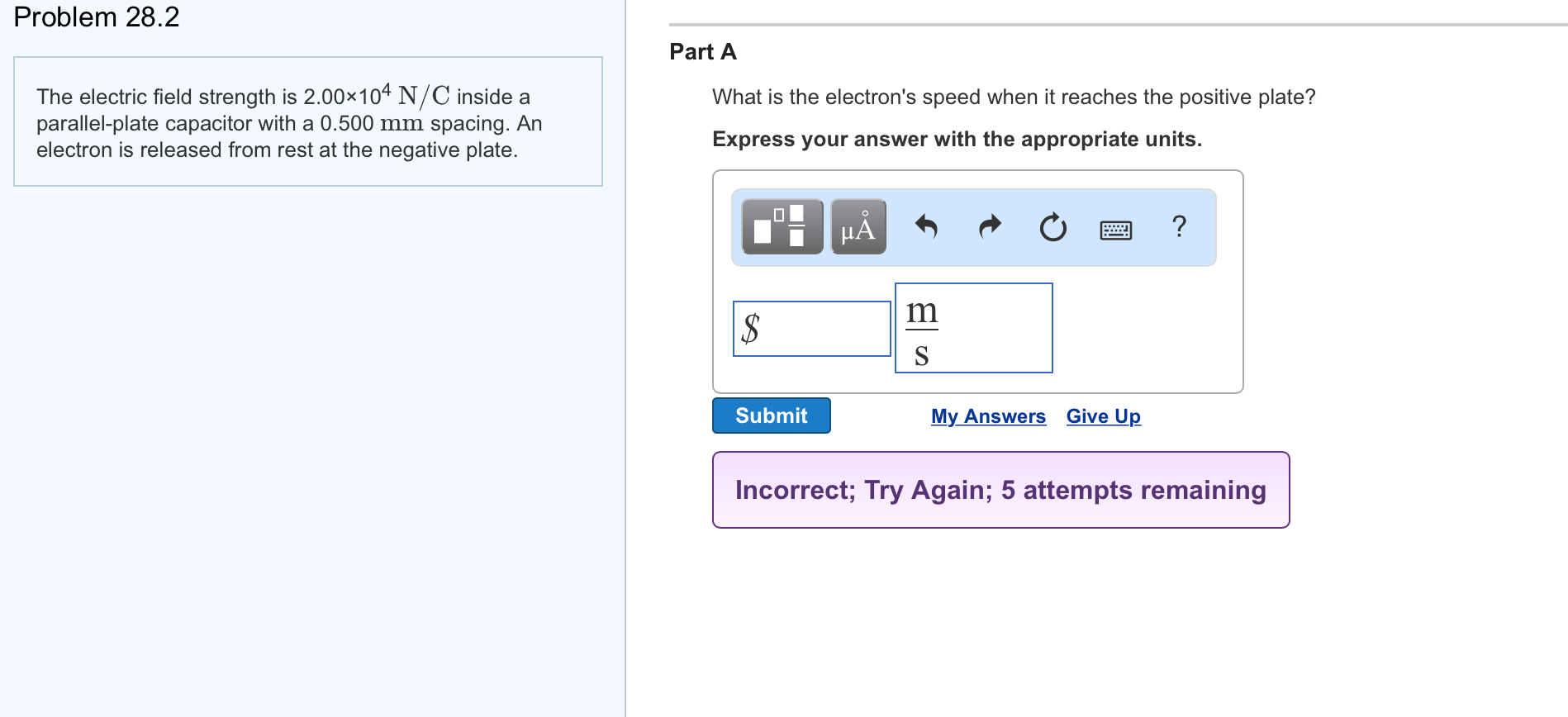The electric field strength is 2.00 times 10^4 N/C inside a parallel-plate capacitor with a 0.500 mm spacing. An electron is released from rest at the negative plate. Part A What is the electron's speed when it reaches the positive plate?

• ### (Connection between E and V in a parallel plate capacitor) We observe that the electric field...

(Connection between E and V in a parallel plate capacitor) We observe that the electric field between the parallel plates of area A, and containing charge Q is uniform, i.e. the E-lines are parallel and E = Q/(εoA). (i) Describe the equipotential lines between the plates. (ii) Draw lines of potential 2.25 V, 4.50 V and 7.25 V between a parallel plate capacitor with potential difference between the plates, ΔVC = 9.00 V

• ### The electric field strength is 5.30x104 N/C inside a parallel-plate capacitor with a 1.70 mm spacing....The electric field strength is 5.30x104 N/C inside a parallel-plate capacitor with a 1.70 mm spacing. A proton is released from rest at the positive plate Part A What is the proton's speed when it reaches the negative plate? Express your answer with the appropriate units. Value Units Submit Request Answer Provide Feedback

• ### The electric field strength is 50,000 N/C inside a parallel plate capacitor with a 2.0 mm spacing

The electric field strength is 50,000 N/C inside a parallel plate capacitor with a 2.0 mm spacing. A proton is released from rest oa the positive plate. What is the proton's speed when it reaches the negative plate?Please show all work, I've got the answer but I want to know how to find it, and I'll figure out why it works from there, and can solve my other questions. Thanks!

• ### An electron is released from rest at the negative plate of a parallel plate capacitor and...

An electron is released from rest at the negative plate of a parallel plate capacitor and accelerates to the positive plate. The plates are separated by a distance of 1.2 cm and the electric field within the capacitor has a magnitude of 2.1 * 10^6 V/m. What is the speed of the electron just as it reaches the positive plate?

• ### An electron is released from rest at the negative plate of a parallel plate capacitor and...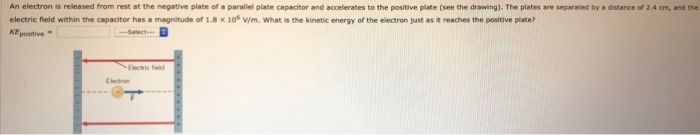An electron is released from rest at the negative plate of a parallel plate capacitor and accelerates to the positive plate (see the drawing). The plates are separated by a distance of 2.4 cm, and the electric field within the capacitor has a magnitude of 1.8 x 10% v/m. What is the kinetic energy of the electron just as it reaches the positive pliate? KEpositive- Electric ield Electron

Free Homework App